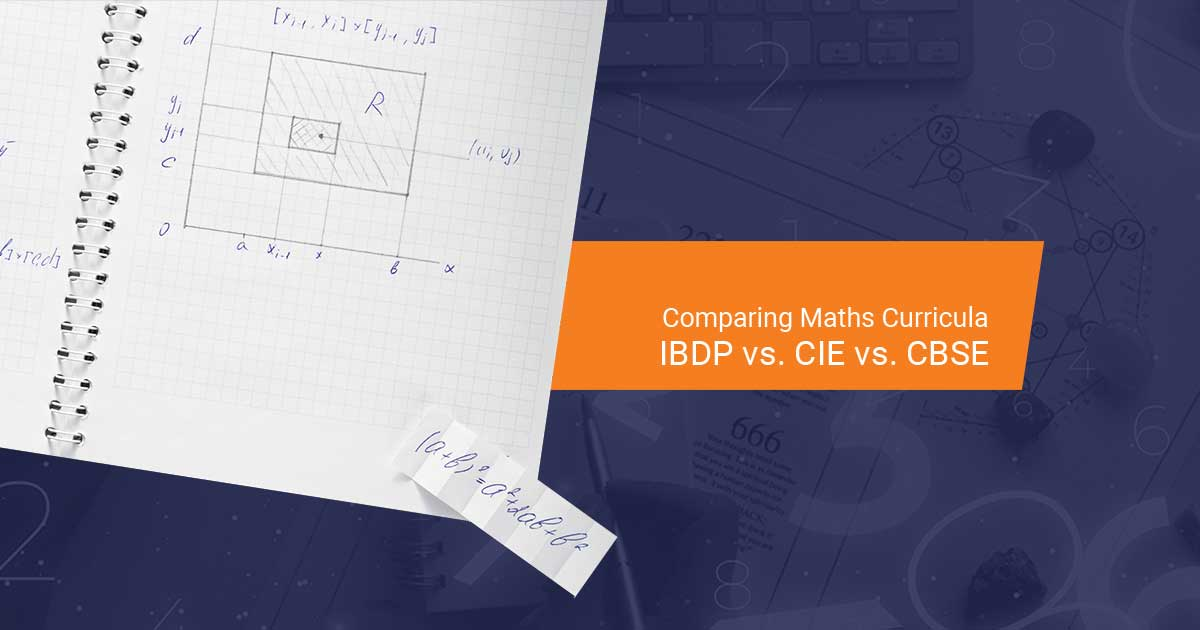##### Comparing Maths Curricula: IBDP vs. CIE vs. CBSE
Oct, 20 2023

Mathematics enables students to dissect complex problems, break them into manageable parts, and develop effective solutions. Furthermore, in an increasingly digital era, mathematics plays a vital role in areas such as computer algorithms, software development, data analysis, and artificial intelligence.

The International Baccalaureate Diploma Programme (IBDP), Cambridge International Examinations (CIE), and the Central Board of Secondary Education (CBSE) offer diverse and comprehensive mathematics curricula tailored to the unique educational needs of students at various stages of their academic journey. Today, we shall explore the distinctive features and key components of the mathematics curricula in the IBDP, CIE, and CBSE in India.

Maths Curriculum in IBDP

Mathematics plays a vital role within the IBDP, covering an extensive syllabus of mathematical principles and their practical application in the real world. The IBDP Mathematics programme is divided into two primary streams: Analysis and Approaches (AA) and Applications and Interpretation (AI). The AA curriculum is focused on nurturing analytical and problem-solving abilities, whereas the AI curriculum emphasises the pragmatic utilisation of mathematical methods. We have summarised the two types of subject combinations included in an IB Maths curriculum:

IB Maths Analysis and Approaches (AA)

IB Math AA is renowned for its focus on abstract and analytical mathematical principles. This syllabus is available in both higher level (HL) and standard level (SL) and is divided into six sections, such as:

• Algebra: Covers fundamental algebraic concepts and equations.
• Functions and Equations: Explores composite and inverse functions, trigonometric functions, and graphs.
• Calculus: Focuses on differentiation, integration, and their applications in analysing rates of change.
• Vectors: Introduces vector operations, scalar and vector products, and their applications in geometry, mechanics, and functions.
• Series and Differential Equations: Discusses series properties, convergence, and divergence.
• Mathematical Induction and Proof: Develops skills in mathematical induction, proof techniques, mathematical logic, and set theory.

IB Maths Applications and Interpretation (AI)

IB Maths AI is also available in HL and SL and emphasises the practical utilisation of mathematical principles in real-life situations. The curriculum equips students with practical mathematical skills for data analysis, mathematical modelling, and problem-solving, such as:

• Statistical Analysis: Focuses on data collection, analysis, probability, and hypothesis testing.
• Financial Mathematics: Applies math to financial scenarios, covering interest, annuities, loans, and investment analysis.
• Mathematical Models: Develop models for real-world phenomena using functions and equations.
• Networks and Decisions Mathematics: Explores network analysis, algorithms, and decision-making.
• Geometry and Trigonometry: Applies geometric and trigonometric principles in fields like surveying and navigation.
• Calculus Applications: Extends calculus concepts to analyse real-world situations in physics, biology, and economics.

Maths Curriculum in CIE

The CIE Maths curriculum fosters the development of essential mathematical skills, serving as a solid foundation for further study of math or the enhancement of skills in other disciplines. The curriculum is designed to enhance the learners' competence, self-assurance, and proficiency in employing mathematical techniques, both with and without calculators. CIE Math also cultivates learners' intuition for quantity, patterns, and relationships, promoting their abilities in reasoning and analysis. The curriculum emphasises developing skills such as problem-solving, both within mathematical contexts and in real-life situations. Following are the key topics that are covered in the CIE Math curriculum:

• Cambridge IGCSE Mathematics: This syllabus covers a wide range of topics in algebra, geometry, statistics, and other areas of mathematics.
• Cambridge International AS and A Level Mathematics: This Cambridge syllabus covers topics from both pure mathematics and applied mathematics components. Pure mathematics encompasses subjects such as calculus and algebra, whereas applied mathematics may cover areas like statistics, mechanics, and decision mathematics.
• Cambridge International AS and A-Level Further Mathematics: The curriculum serves as an extension of A-Level Mathematics, catering to students seeking a deeper exploration of advanced mathematical concepts. The syllabus covers areas such as complex numbers, matrices, differential equations, and more.
• Cambridge Pre-U Mathematics: The Cambridge Pre-U is an alternative qualification to A Levels and covers topics from pure mathematics and statistics.
• Cambridge O-Level Additional Mathematics: This is an optional subject that can be taken in addition to Cambridge IGCSE Mathematics. The syllabus covers more advanced topics such as calculus and trigonometry.

Maths Curriculum in CBSE

The CBSE Math curriculum is designed to provide a comprehensive understanding of mathematics principles and theories for students from primary to secondary levels. Here is an overview of the CBSE mathematics curriculum at various levels:

• Primary Level (Classes 1 to 5): The primary focuses on the development of fundamental mathematical skills. The topics typically addressed at this level consist of numbers, elementary arithmetic operations (addition, subtraction, multiplication, and division), measurement, time, currency, geometry, and fundamental fractions.
• Middle Level (Classes 6 to 8): The middle stage introduces more advanced mathematical concepts. Students often learn topics such as algebra, geometry, mensuration, statistics, and probability. In addition to these subjects, students develop an understanding of integers, rational numbers, algebraic expressions, linear equations, and various others.
• Senior Secondary Level (Classes 11 and 12): As for the secondary level (Classes 9 and 10), the math curriculum encompasses subjects like real numbers, polynomials, coordinate geometry, linear equations, quadratic equations, trigonometry, statistics, and probability. The syllabus is further bifurcated into two levels: Mathematics Standard (Maths) and Mathematics Basic (Basic Maths).

Final Thoughts

The mathematics curricula in the IBDP, CIE, and CBSE offer unique pathways for students to develop knowledge and skills related to mathematical principles and applications. The IBDP's AA and AI, CIE's, and CBSE’s math curricula are tailored to enable students to develop mathematical competencies for both academic excellence and real-world problem-solving. Though the choice of the curriculum depends on the educational system students are part of, each curriculum contributes significantly to the development of mathematical proficiency and critical thinking skills.